# e. public class Queue { // Uses the correct Stack class from ME2 Ex 19 private Stack mStack; public Queue() { setStack(new Stack()); } public Queue enqueue(E pData) { getStack().push(pData); return t... related homework questions

• #### e. public class Queue { // Uses the correct Stack class from ME2 Ex 19 private Stack mStack; public Queue() { setStack(new Stack()); } public Queue enqueue(E pData) { getStack().push(pData); return t...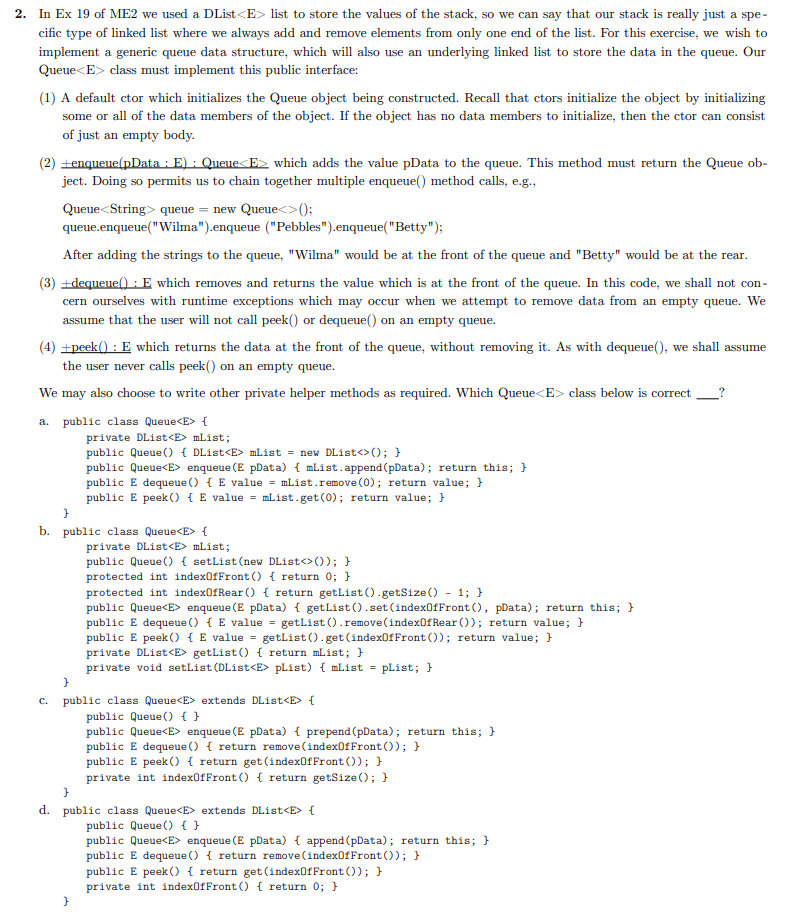e. public class Queue { // Uses the correct Stack class from ME2 Ex 19 private Stack mStack; public Queue() { setStack(new Stack()); } public Queue enqueue(E pData) { getStack().push(pData); return this; } public E dequeue() { return getStack().pop(); } public E peek() { return getStack.peek(); } private Stack getStack() { return mStack; } private void setStack(Stack pStack) { mStack...

• #### It’s review question, I need this as soon as possible. Thank you 3) For thè diferential equation: (a) The point zo =-1 is an ordinary point. Compute the recursion formula for the coefficients of...It’s review question, I need this as soon as possible. Thank you 3) For thè diferential equation: (a) The point zo =-1 is an ordinary point. Compute the recursion formula for the coefficients of the power series solution centered at zo- -1 and use it to compute the first three nonzero terms of the power series when -1)-s and v(-1)-0....

• #### Dont copié formé thé book oh ya dont copié formé thé book cause you Oiil inde up being triste soi remembré not toi copié frome thé book oh ya

Dont copié formé thé book oh ya dont copié formé thé book cause you Oiil inde up being triste soi remembré not toi copié frome thé book oh ya!translation in english please!

• #### JAVA PROGRAM: public class Stack { private DList mList; public Stack() { setList(new DList<>()); } private int indexOfTop() { return getList().isEmpty() ? -1 : getList().getSize() - 1; } public...

JAVA PROGRAM: public class Stack { private DList mList; public Stack() { setList(new DList<>()); } private int indexOfTop() { return getList().isEmpty() ? -1 : getList().getSize() - 1; } public E peek() { return getList().get(indexOfTop()); } public E pop() { return getList().remove(indexOfTop()); } public Stack push(E pData) { getList().append(pData); return this; } private DList getList() { return mList; } private void...

• #### Create a flowchart to represent the Push and Pop operations for a Stack based on a linked list data structure. Create a flowchart to represent the Enqueue and Dequeue operations for a Queue based on a...

Create a flowchart to represent the Push and Pop operations for a Stack based on a linked list data structure. Create a flowchart to represent the Enqueue and Dequeue operations for a Queue based on a linked list data structure. Write the required Java code to implement either a Stack or a Queue data structure based on a linked list....

• #### Write Junit Test for the following class below: public class Turn {    private int turnScore;    private Dice dice;    private boolean isSkunk;    private boolean isDoubleSkunk;    public Turn()    {...

Write Junit Test for the following class below: public class Turn {    private int turnScore;    private Dice dice;    private boolean isSkunk;    private boolean isDoubleSkunk;    public Turn()    {        dice = new Dice();    }    public Turn(Dice dice) {        this.dice = dice;    }       public void turnRoll()    {        dice.roll();        if (dice.getDie1Value() == 1 || dice.getDie2Value() == 1 && dice.getLastRoll() != 2)        {            turnScore =...

• #### template <class T> class Stack { public: /** clear * Method to clear out or empty any items on stack, * put stack back to empty state. * Postcondition: Stack is empty. */ virtual void clear() =...

template <class T> class Stack { public: /** clear * Method to clear out or empty any items on stack, * put stack back to empty state. * Postcondition: Stack is empty. */ virtual void clear() = 0; /** isEmpty * Function to determine whether the stack is empty. Needed * because it is undefined to pop from empty stack....

• #### DSuppose \$39oo is deposited in a savings account that increases exponentially.Detamine thě APv if the acount...DSuppose \$39oo is deposited in a savings account that increases exponentially.Detamine thě APv if the acount increases to \$t020 in 4 years. Ass ume tne interest Vale remains Constant and no additional deposits or Withdrawals are made. (a.) Let pbe the APY. Note tnat if tme inital balaqe is yo, ne year later tne balane is %more. P- 3 (Tpe...

• #### AQueue.java class AQueue implements Queue { private E queueArray[]; // Array holding queue elements private static...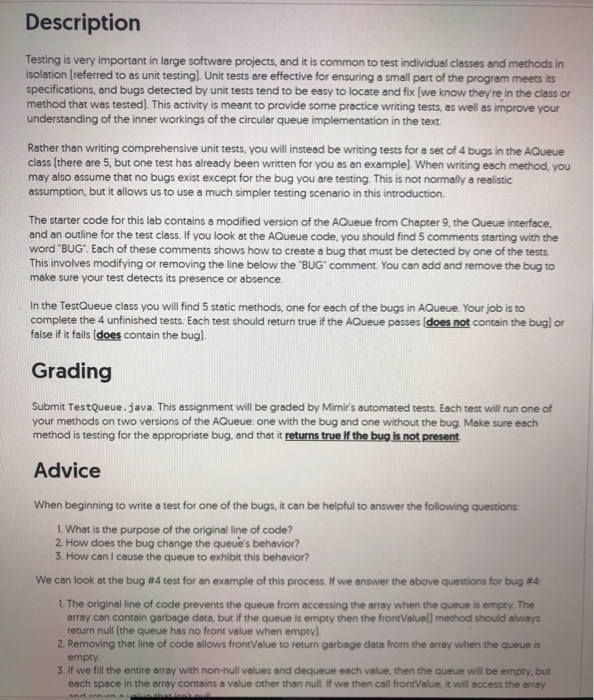AQueue.java class AQueue implements Queue { private E queueArray[]; // Array holding queue elements private static final int DEFAULT_SIZE = 10; private int maxSize; // Maximum size of queue private int front; // Index of front element private int rear; // Index of rear element // Constructors @SuppressWarnings("unchecked") // Generic array allocation AQueue(int size) { //BUG #1: maxSize = size...

• #### skeleton: import java.util.*; public class Point3D{ private int x,y,z; Point3D(int a, int b, int c){ x = a; y = b; z = c; } public int x(){ return x; } public int y(){ return y; } public in...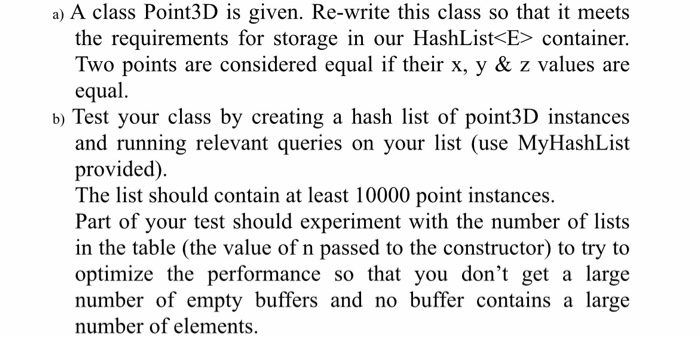skeleton: import java.util.*; public class Point3D{ private int x,y,z; Point3D(int a, int b, int c){ x = a; y = b; z = c; } public int x(){ return x; } public int y(){ return y; } public int z(){ return z; } public String toString(){ return "("+x+","+y+","+z+")"; } } Question1Test.java import java.util.*; public class Question1Test { public static void...

• #### Create Junit tests for the following classes public class Dice {    private Die die1;    private Die die2;    public Dice()    {        this.die1 = new Die();        this.die2 = new Die();    }    pu...

Create Junit tests for the following classes public class Dice {    private Die die1;    private Die die2;    public Dice()    {        this.die1 = new Die();        this.die2 = new Die();    }    public Dice(int[] programmedRolls)    {        int[] programmableroll = programmedRolls;        this.die1 = new Die(programmableroll);        this.die1 = new Die(programmableroll);           }       public Dice(Die die1, Die die2)    {        this.die1 = die1;        this.die2 = die2;    }       public...

• #### Please write algorithm and IPO for the following JAVA code using microsoft word... public class Party {    // Declaring instance variables    private int guests;    private String date;    private Str...

Please write algorithm and IPO for the following JAVA code using microsoft word... public class Party {    // Declaring instance variables    private int guests;    private String date;    private String time;    // Zero argumented constructor    public Party() {        this.guests = 50;        this.time = "6:00 PM";    }    // Parameterized constructor    public Party(int guests, String time) {        this.guests = guests;        this.time = time;   ...

• #### Write JunitTest for the class below: public class Dice {    private Die die1;    private Die die2;    public Dice(int[] programmedRolls)    {        int[] programmableroll = programmedRolls;        th...

Write JunitTest for the class below: public class Dice {    private Die die1;    private Die die2;    public Dice(int[] programmedRolls)    {        int[] programmableroll = programmedRolls;        this.die1 = new Die(programmableroll);        this.die1 = new Die(programmableroll);    } }

• #### Write Junit test for the following class below: public class Player {       public int turnScore;    public int roundScore;    public int lastTurnScore;    public String name;    public int chipPile;...

Write Junit test for the following class below: public class Player {       public int turnScore;    public int roundScore;    public int lastTurnScore;    public String name;    public int chipPile;       public Player (String name) {        this.name = name;        this.turnScore = 0;        this.chipPile = 50;    }          public int getChip() {        return chipPile;    }       public void addChip(int chips) {        chipPile += chips;    }       public int...

• #### Consider the following class definition class FibSequence implements Iterablexnteger public Iterator<Integer> iterator) return new FibIterator); private class FibIterator implements Iterator&lt...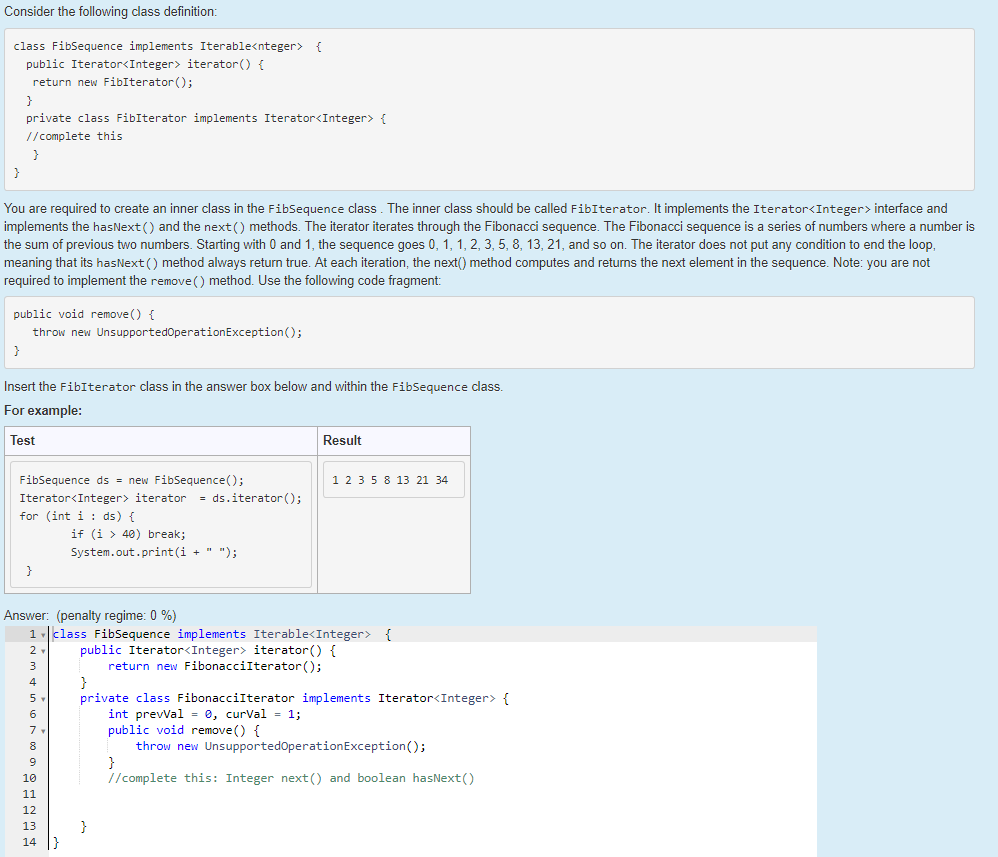Consider the following class definition class FibSequence implements Iterablexnteger public Iterator<Integer> iterator) return new FibIterator); private class FibIterator implements Iterator<Integer> f //complete this You are required to create an inner class in the FibSequence class. The inner class should be called FibIterator. It implements the Iterator<Integer> interface and implements the hasNext() and the next() methods. The iterator iterates through the...

• #### in python and according to this #Creating class for stack class My_Stack: def __init__(self): self.items = [] def isEmpty(self): return self.items == [] def Push(self, d): self.items.append(d) def Po...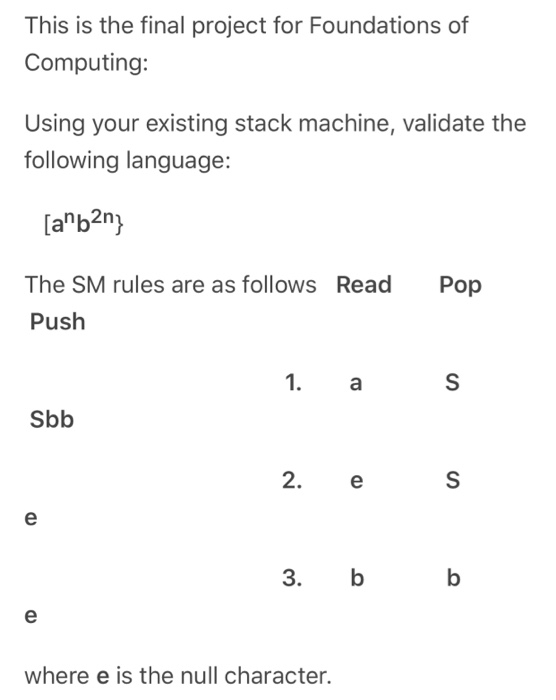in python and according to this #Creating class for stack class My_Stack: def __init__(self): self.items = [] def isEmpty(self): return self.items == [] def Push(self, d): self.items.append(d) def Pop(self): return self.items.pop() def Display(self): for i in reversed(self.items): print(i,end="") print() s = My_Stack() #taking input from user str = input('Enter your string for palindrome checking: ') n= len(str) #Pushing half of...

• #### Answer this plz with java lang. Question is : Here is the class you will use public interface Stack<E> {    /**    * Returns the number of elements in the stack.    * @return number of elements...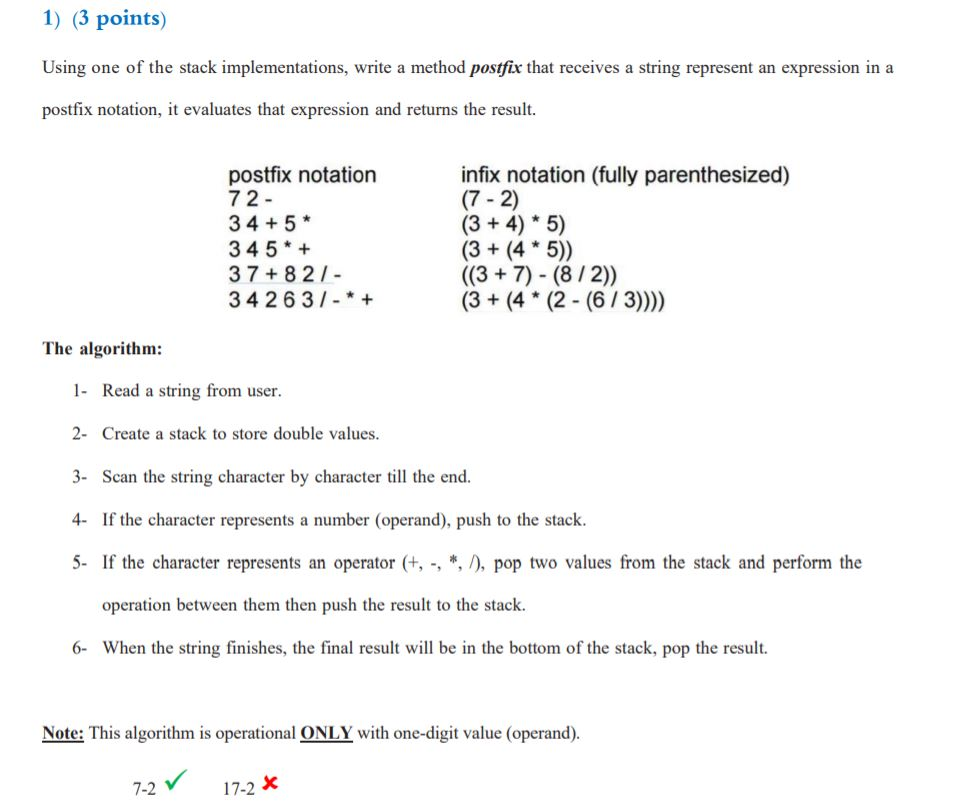Answer this plz with java lang. Question is : Here is the class you will use public interface Stack<E> {    /**    * Returns the number of elements in the stack.    * @return number of elements in the stack    */    int size( );    /**    * Tests whether the stack is empty.    * @return true if the stack is empty, false otherwise   ...

• #### public class ConsCell { private int head; private ConsCell tail; public ConsCell(int h, ConsCell t) {...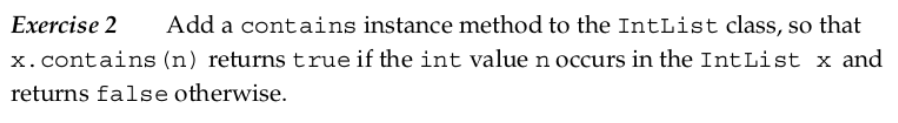public class ConsCell { private int head; private ConsCell tail; public ConsCell(int h, ConsCell t) { head = h; tail = t; } public int getHead() { return head; } public ConsCell getTail() { return tail; } public void setTail(ConsCell t) { tail = t; } } public class IntList { private ConsCell start; public IntList (ConsCell s) { start...

• #### public class Point f private int x; private int y; public Point(int x, int y) this.x...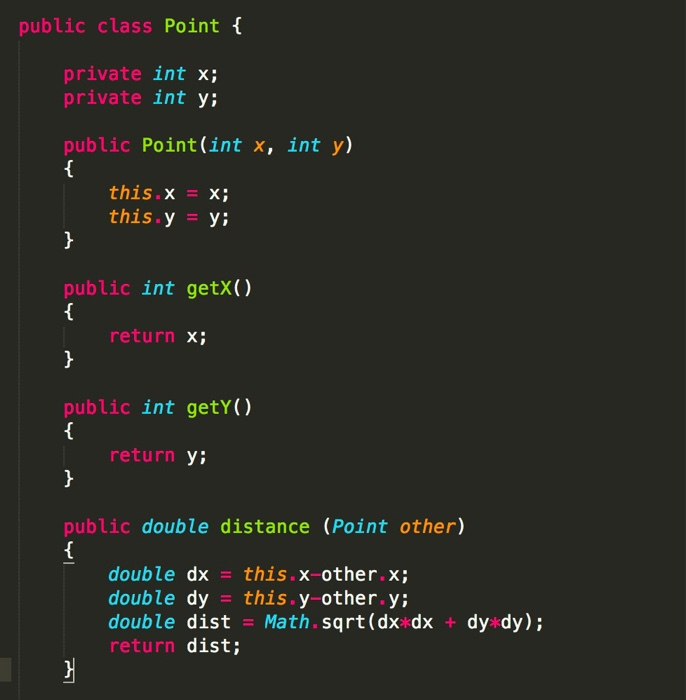public class Point f private int x; private int y; public Point(int x, int y) this.x X; this.y y; public int getX() return x; public int getY() return Y: public double distance (Point other) double dx this.x-other.x; double dy this.y-other.y double dist Math.sqrt(dx dx + dy dy); return dist;

Need Online Homework Help?